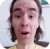FreeKB - Perl Getting Started with JSON::Parse
Perl - Getting Started with JSON::ParseThe JSON::Parse module can be used to parse json.

``use JSON::Parse 'parse_json';``

For example, let's say you have the following JSON.

``````{
"foo_key": "Hello",
"bar_key": "World"
}
``````

In this example, the JSON is stored in a variable named raw_json.

``my \$raw_json = '{"foo_key":"Hello", "bar_key":"World"}';``

Or, if the JSON is in a file, you would do the following to store the JSON in a variable named raw_json.

``````local \$/;
open(FH, "<:encoding(UTF-8)", "/path/to/example.json") or die "cannot open example.json \$! \n";
my \$raw_json = <FH>;
close FH;``````

Attempting to print the variable . . .

``print \$raw_json;``

... with print either ARRAY ...

``ARRAY``

... or HASH.

``HASH``

You will want to store the parsed json in a new variable.

``my \$parsed_json = parse_json(\$raw_json);``

Dumper can be used to print the parsed json.

``````use Data::Dumper;
print Dumper \$parsed_json;``````

Dumper should produce something like this. There are no [ ] brackets, thus it's a hash.

``````\$VAR1 = {
'foo_key' => 'Hello',
'bar_key' => 'World'
};
``````

Or like this. There are [ ] brackets, thus it's an array.

``````\$VAR1 = [
{
'foo_key' => 'Hello',
'bar_key' => 'World'
}
];
``````

If it's a hash, the following will print the value in foo_key.

``print \$parsed_json->{foo_key};``

Which should print Hello.

``Hello``

If it's an array, the following will print the value in foo_key.

``````foreach my \$line (@{\$parsed_json}) {
print \$line->{foo_key};
}``````

Which should print Hello.

``Hello``

You can then loop through each key/value pair in the JSON file and print each key.

``````foreach my \$line (@{\$parsed_json}) {

foreach my \$key (keys(%{\$line})) {
print \$key;
}

foreach my \$value (values(%{\$line})) {
print \$value;
}

}``````

The print \$key line will print each key, which is just foo_key and bar_key in this example.

``````foo
bar``````

The print \$value line will print each value.

``````Hello
World``````

Instead of looping through each value, you can just get the value from the key.

``````foreach my \$line (@{\$parsed_json}) {

foreach my \$key (keys(%{\$line})) {
print \$key;
}

my \$value = \$key->{foo_key};
print \$value;

}``````

We will never share your name or email with anyone. Enter your email if you would like to be notified when we respond to your comment.

Please enter 6b054 in the box below so that we can be sure you are a human.

Web design by yours truely - me, myself, and I   |   jeremy.canfield@freekb.net   |